Скачать презентацию Macroeconomic Implications of the Exchange Rate Theory Based

• Количество слайдов: 13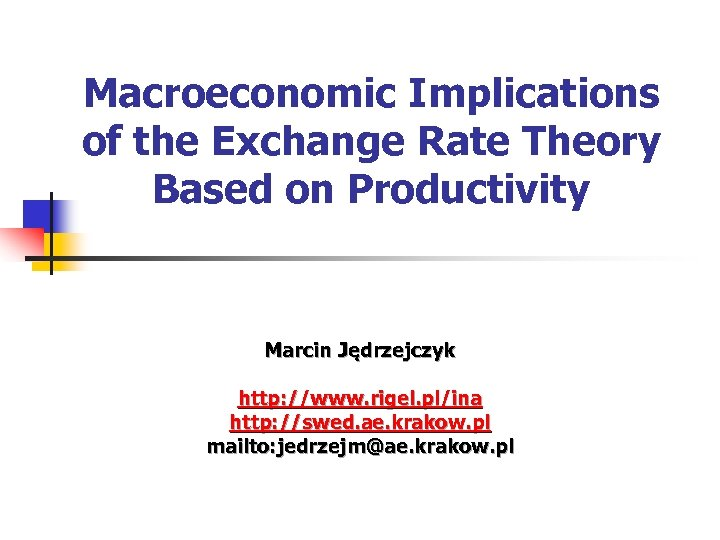Macroeconomic Implications of the Exchange Rate Theory Based on Productivity Marcin Jędrzejczyk http: //www. rigel. pl/ina http: //swed. ae. krakow. pl mailto: [email protected] krakow. pl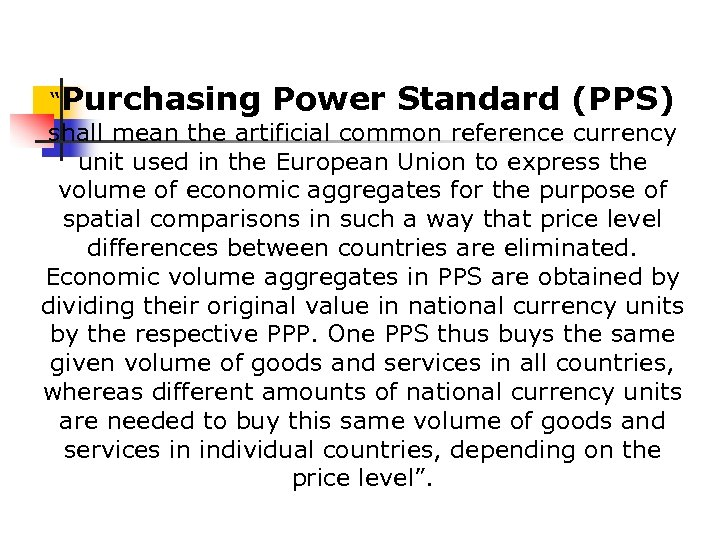“Purchasing Power Standard (PPS) shall mean the artificial common reference currency unit used in the European Union to express the volume of economic aggregates for the purpose of spatial comparisons in such a way that price level differences between countries are eliminated. Economic volume aggregates in PPS are obtained by dividing their original value in national currency units by the respective PPP. One PPS thus buys the same given volume of goods and services in all countries, whereas different amounts of national currency units are needed to buy this same volume of goods and services in individual countries, depending on the price level”.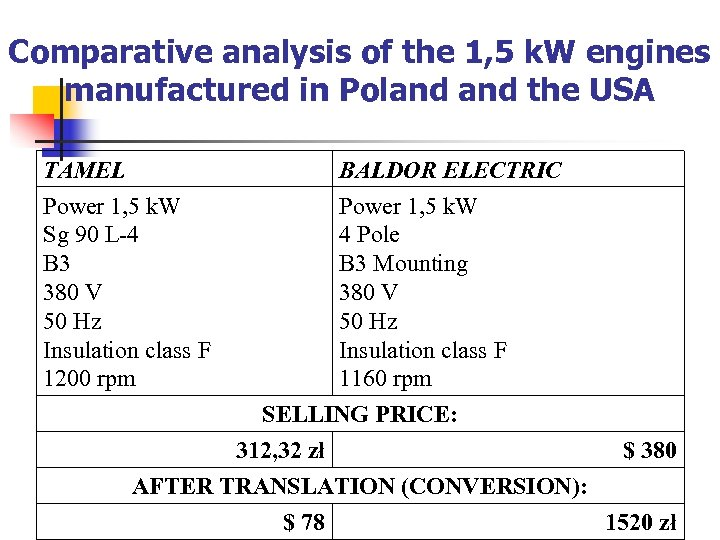Comparative analysis of the 1, 5 k. W engines manufactured in Poland the USA TAMEL Power 1, 5 k. W Sg 90 L-4 B 3 380 V 50 Hz Insulation class F 1200 rpm BALDOR ELECTRIC Power 1, 5 k. W 4 Pole B 3 Mounting 380 V 50 Hz Insulation class F 1160 rpm SELLING PRICE: 312, 32 zł \$ 380 AFTER TRANSLATION (CONVERSION): \$ 78 1520 złPURCHASING POWER STANDARD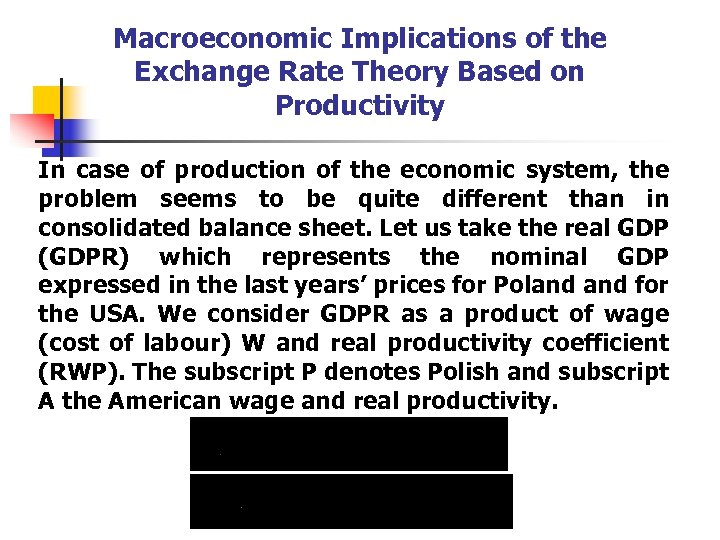Macroeconomic Implications of the Exchange Rate Theory Based on Productivity In case of production of the economic system, the problem seems to be quite different than in consolidated balance sheet. Let us take the real GDP (GDPR) which represents the nominal GDP expressed in the last years’ prices for Poland for the USA. We consider GDPR as a product of wage (cost of labour) W and real productivity coefficient (RWP). The subscript P denotes Polish and subscript A the American wage and real productivity.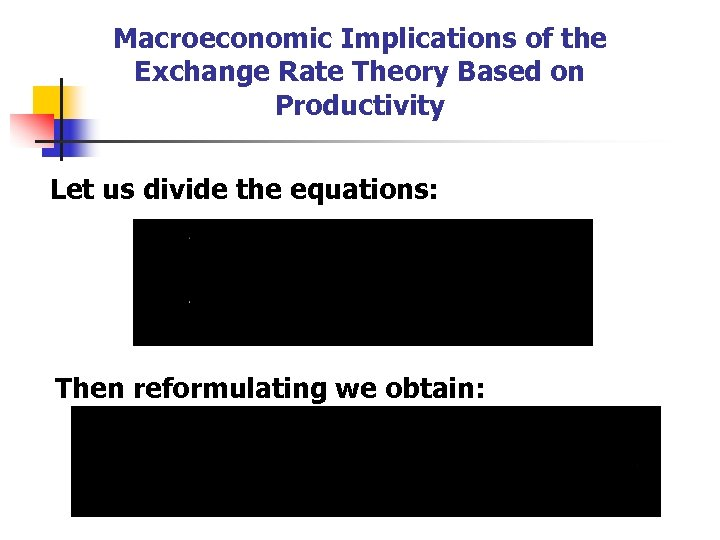Macroeconomic Implications of the Exchange Rate Theory Based on Productivity Let us divide the equations: Then reformulating we obtain: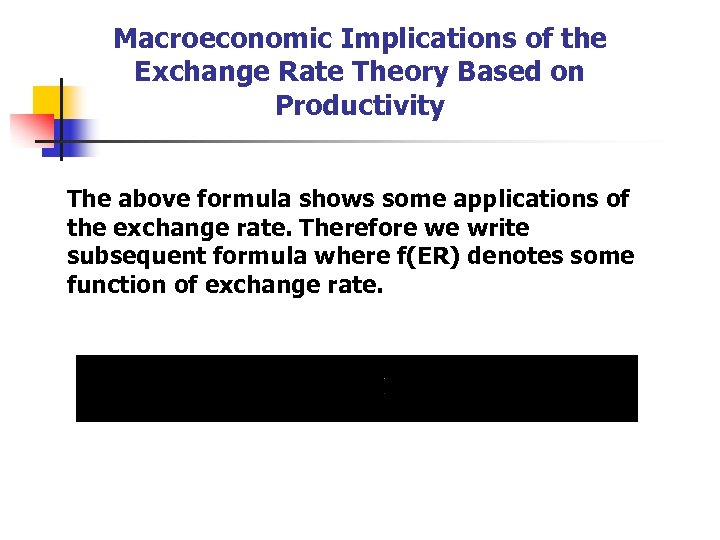Macroeconomic Implications of the Exchange Rate Theory Based on Productivity The above formula shows some applications of the exchange rate. Therefore we write subsequent formula where f(ER) denotes some function of exchange rate.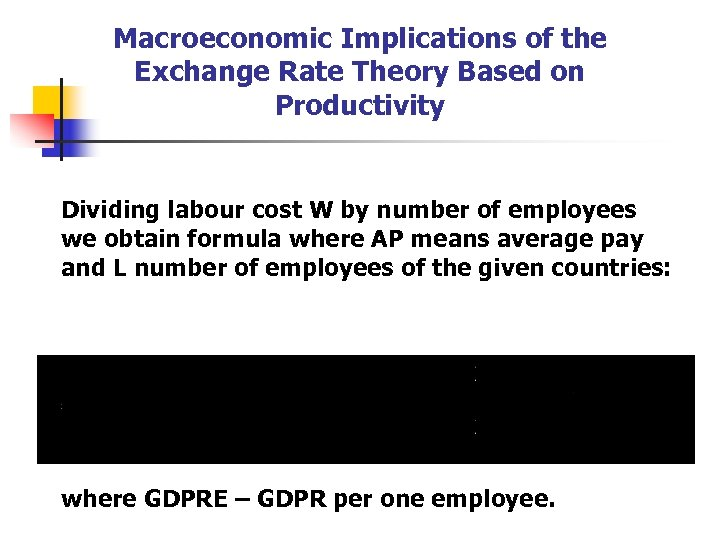Macroeconomic Implications of the Exchange Rate Theory Based on Productivity Dividing labour cost W by number of employees we obtain formula where AP means average pay and L number of employees of the given countries: where GDPRE – GDPR per one employee.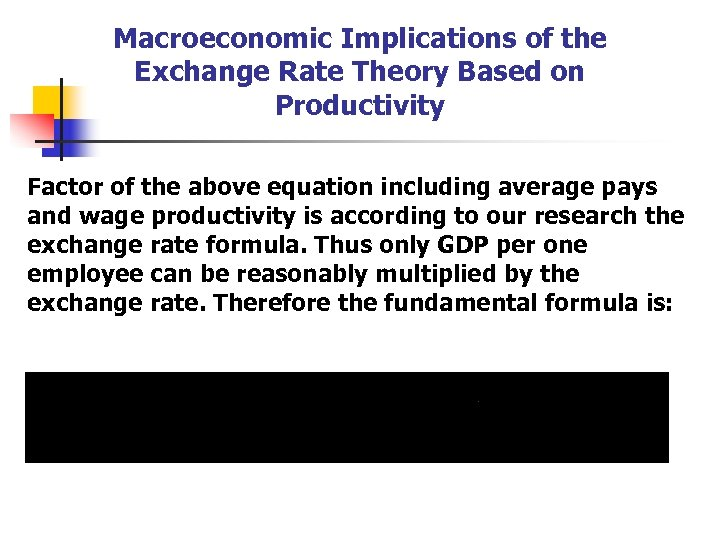Macroeconomic Implications of the Exchange Rate Theory Based on Productivity Factor of the above equation including average pays and wage productivity is according to our research the exchange rate formula. Thus only GDP per one employee can be reasonably multiplied by the exchange rate. Therefore the fundamental formula is: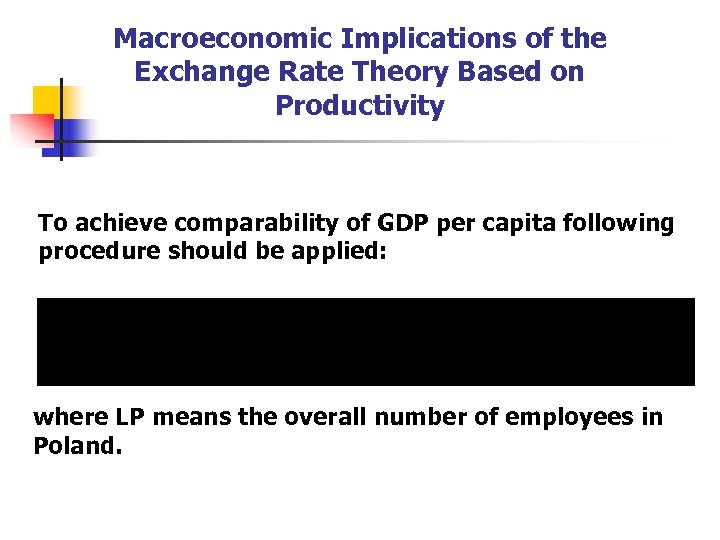Macroeconomic Implications of the Exchange Rate Theory Based on Productivity To achieve comparability of GDP per capita following procedure should be applied: where LP means the overall number of employees in Poland.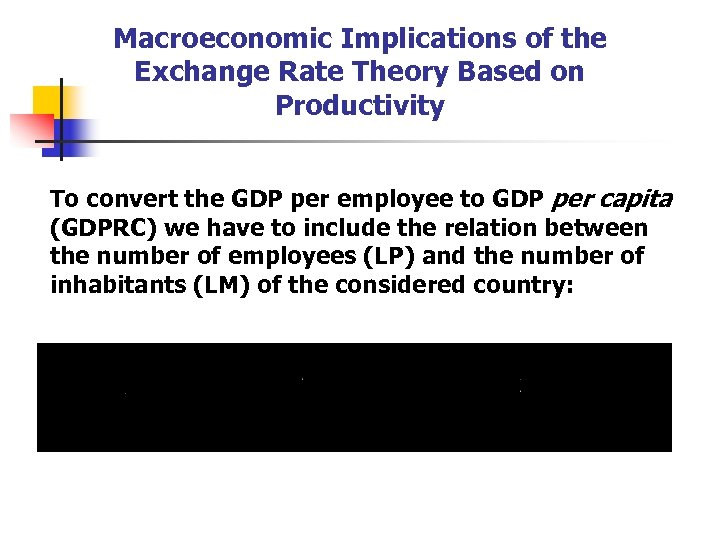Macroeconomic Implications of the Exchange Rate Theory Based on Productivity To convert the GDP per employee to GDP per capita (GDPRC) we have to include the relation between the number of employees (LP) and the number of inhabitants (LM) of the considered country: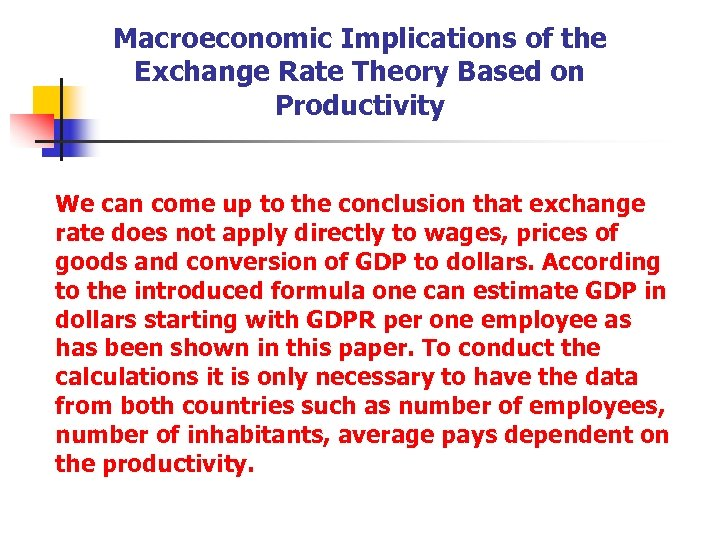Macroeconomic Implications of the Exchange Rate Theory Based on Productivity We can come up to the conclusion that exchange rate does not apply directly to wages, prices of goods and conversion of GDP to dollars. According to the introduced formula one can estimate GDP in dollars starting with GDPR per one employee as has been shown in this paper. To conduct the calculations it is only necessary to have the data from both countries such as number of employees, number of inhabitants, average pays dependent on the productivity.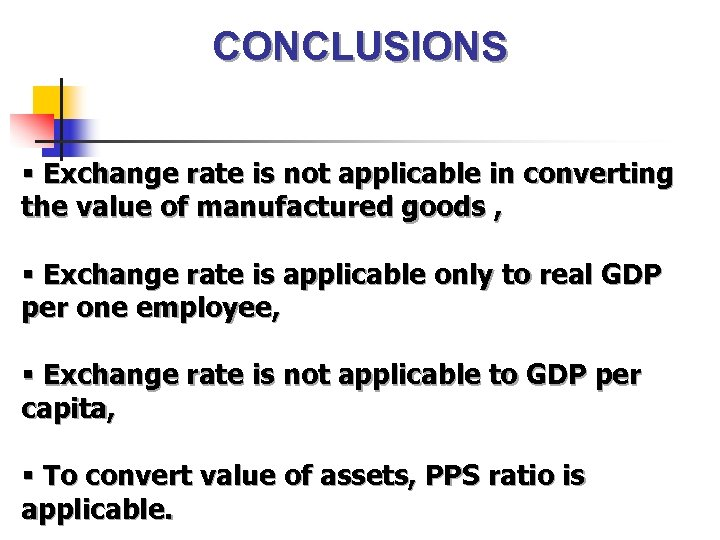CONCLUSIONS § Exchange rate is not applicable in converting the value of manufactured goods , § Exchange rate is applicable only to real GDP per one employee, § Exchange rate is not applicable to GDP per capita, § To convert value of assets, PPS ratio is applicable.